## Walking Columns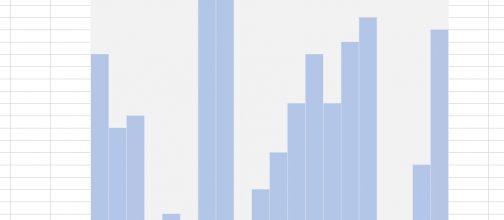An easy way to scroll through set of data in a graphic way without graphs. A-pure-formula xlsx workbook. These were done using formulas, conditional formatting, and ActiveX control.

## Repeated Offset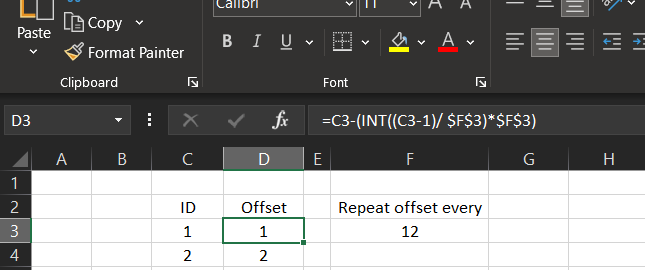I needed to have an offset of columns to be repeated every 12 months. This is part of a project, we needed to calculate the coefficient of sales prediction based …

## Get column name (columnname as A,B,C, etc) as input inside cell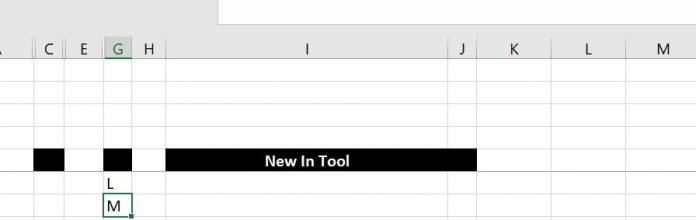Excel already has the formula ROW() =Row() Which as we may already know will give us the number of the row we are in, if you add no parameters However, …

## iframe in Excel (XLiFrame)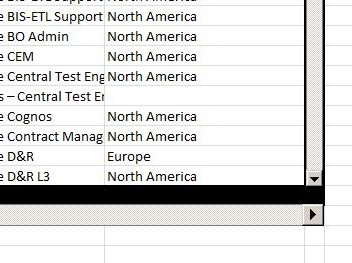This is the iframe in Excel, if you are familiar with the HTML concept of iframe, you will understand this one right away, it is basically the same project as …

## Rotate table using 1 formula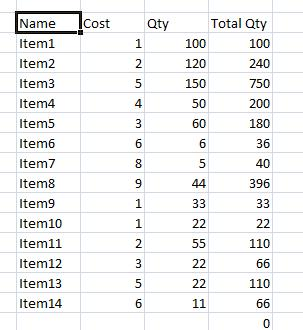Rotating table (Transpose) using functions so that the table is updated once the source table is updated. Also can be used as automatically rotating tables with variable number of rows …

## Sort list dynamically (functions)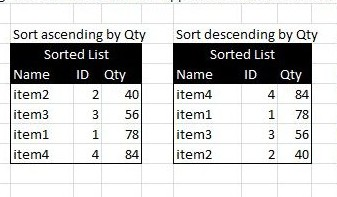Sorting a list automatically using formulas, with no need to press the sort command Also if the source table is changed, the destination table will do also. What you need …

## Insert Blank rows into table using functions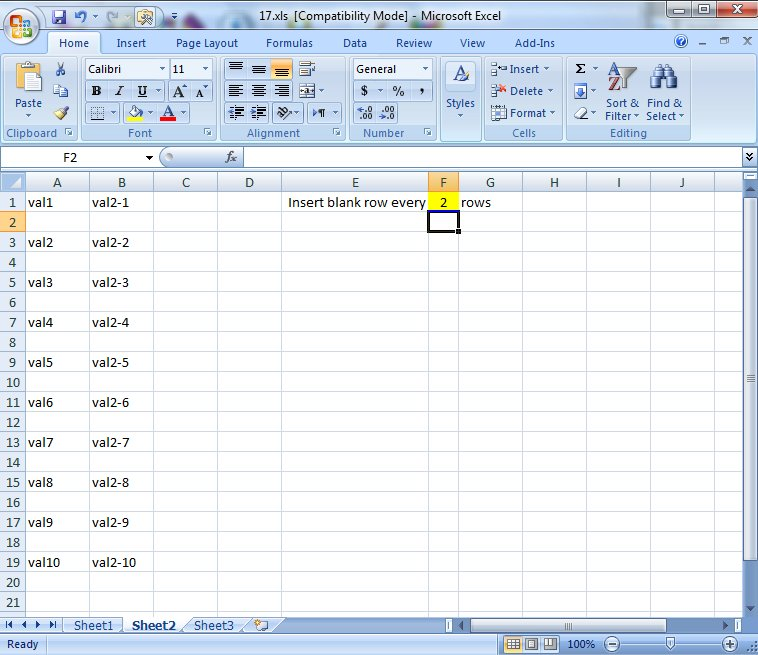Here you will see how to work with functions to insert a blank row every certain number of rows in a table using a blank sheet to copy all values …